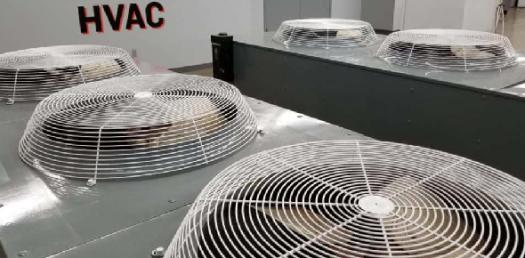# HVAC Controls Trivia Questions! Quiz

27 Questions | Attempts: 304
ShareSettingsHVAC Controls Final Quiz

• 1.
The control result is not monitored for which system? (i.e. The output variable xa of the controlled system has no backward effect on the controlling equipment)
• A.

Open Loop

• B.

Closed Loop

• 2.
A humidification sequence would use what type of controller?
• A.

Direct acting

• B.

Reverse Acting

• 3.
HVAC systems are almost always:
• A.

Non self-regulating (astatic or integral system, I-systems)

• B.

Self-regulating (static or proportional systems, P-systems)

• 4.
The Transfer Coefficient of the Controlled System is represented by
• A.

Tn

• B.

Tv

• C.

Ks

• D.

Kd

• E.

Xh

• 5.
If the control range Xh is too large, then...
• A.

The setpoint can't be achieved if the maximum disturbance variable occurs

• B.

The control loop tends to oscillate

• 6.
In PT1, the 1 refers to:
• A.

One storage element

• B.

One control system

• C.

One slave controller

• 7.
The controllability of a system ___________ with an increasing number of storage elements.
• A.

Increases

• B.

Decreases

• C.

Doesn't change

• 8.
Which of the following is not a way to describe the dynamic response of a controlled system?
• A.

Description via step response

• B.

Description by the Ziegler-Nichols method

• C.

Description by the control variable response

• D.

Description via the frequency response (harmonic response)

• 9.
Information on how the controlled system reacts to a sudden increase in heat gains would be best provided by:
• A.

Manipulated variable step response

• B.

Disturbance variable step response

• 10.
The "correcting span" is:
• A.

Xp

• B.

Xh

• C.

Yh

• D.

Tv

• 11.
The main storage element in an electrically heated hot water tank is the:
• A.

Electric resistance element

• B.

Water

• C.

Agitator

• D.

Thermometer

• E.

Tank Insulation

• 12.
Is dead time the same as travel time?
• A.

Yes

• B.

No

• 13.
If there are a lot of storage elements in the system, the step response:
• A.

Has a steeper slope

• B.

Has a gentler slope

• 14.
Tu, Tg, and Ts are (in order)
• A.

Balancing Time, Delay Time, Time Constant

• B.

Time Constant, Balancing Time, Delay Time

• C.

Delay Time, Time Constant, Balancing Time

• D.

Delay Time, Balancing Time, Time Constant

• 15.
The degree of difficulty S (Tu/Tg) represents:
• A.

The control range of a control system

• B.

The controllability of a controlled system

• C.

The reaction time of a controlled system

• 16.
A difficult-to-control system has a degree of difficulty (S) of:
• A.

S

• B.

S=0.1...0.3

• C.

S>0.3

• 17.
Controlled systems in the ventilation field tend to have ______degrees of difficulty and _________ balancing times when compared to the heating field.
• A.

Higher, longer

• B.

Higher, shorter

• C.

Lower, longer

• D.

Lower, shorter

• 18.
The oscillation test (Ziegler-Nichols method) is performed in what environment?
• A.

Closed Loop

• B.

Open Loop

• 19.
________ controllers are mainly used for temperature, pressure, or level control.
• A.

Powered controllers

• B.

Self-actuated controllers

• 20.
A direct-acting controller only activates its output if e is
• A.

Less than 0

• B.

Greater than 0

• 21.
Which controller type can position its output variable at two or more pre-defined values within the entire correcting span Yh?
• A.

Two-position

• B.

Modulating

• C.

Non-modulating

• D.

Quasi-modulating

• 22.
The smaller the proportional band Xp that is selected, the __________________ the offset will be, but the ______ the tendency of the control loop to oscillate.
• A.

Larger, smaller

• B.

Smaller, greater

• 23.
True or false: the P-controller is load-independent and the I-controller is load-dependent.
• A.

True

• B.

False

• 24.
A two-position controller would be a good choice for all but which of the following applications?
• A.

As a hygrostat to switch the humidifier of a room humidity control system

• B.

As a pressostat to switch the compressor of a compressed air station

• C.

As a flow temperature low-limit controller in a floor heating system

• D.

As a temperature controller for a domestic hot water heater

• E.

As a frost protection thermostat in a ventilation system

• 25.
A generally applied rule of thumb recommends that the loop gain Vo should be set to __________ of the critical loop gain.
• A.

One-fourth

• B.

Half

• C.

Three-fourths

## Related TopicsBack to top
×

Wait!
Here's an interesting quiz for you.